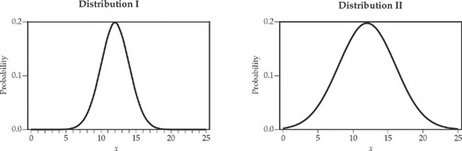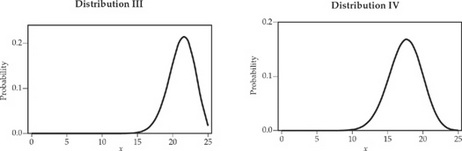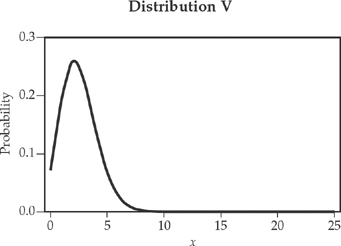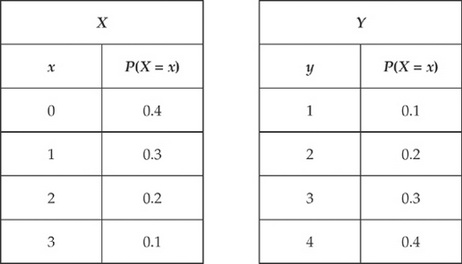# AP Statistics Practice Test 3

### Test Information10 questions23 minutes

1. Two dice are rolled simultaneously. If both dice show 6, then the player wins \$20; otherwise the player loses the game. It costs \$2.00 to play the game. What is the expected gain or loss per game?

2. According to the central limit theorem, the sample mean X is approximately normally distributed

3. A department store at a large mall claims that over 60 percent of the mall’s visitors shop at that store. Let p = the proportion of the mall’s visitors who shop at the store. Which of the following pairs of hypotheses should be used to support this claim?

4. A random sample of 16 light bulbs of one brand was selected to estimate the mean lifetime of that brand of bulbs. The sample mean was 1,025 hours, with a standard deviation of 130 hours. Assuming that the lifetimes are approximately normally distributed, which of the following will give a 95 percent confidence interval to estimate the mean lifetime?

5. A school committee member is lobbying for an increase in the gasoline tax to support the county school system. The local newspaper conducted a survey of county residents to assess their support for such an increase. What is the population of interest here?

6. A manufacturer of ready-bake cake mixes is interested in designing an experiment to test the effects of four different temperature levels (300, 325, 350, and 375F), two different types of pans (glass and metal), and three different types of ovens (gas, electric, and microwave) on the texture of its cakes, in all combinations. Which of the following below is the best description of the design of the necessary experiment?

7. Which one of the following distributions could have a mean of approximately 12 and a standard deviation of approximately two?8. The relation between the selling price of a car (in \$1,000) and its age (in years) is estimated from a random sample of cars of a specific model. The relation is given by the following formula:

Selling price = 15.9 – 0.983 (age)

Which of the following can we conclude from this equation?

9. Which of the following is true about any discrete probability distribution of a random variable X?

10.X and Y are independent random variables; the probability distributions for each are given in the table above. Let Z = X + Y. What is P(Z ≤ 2)?